# Higher derivative

## Definition

### Terminology

Higher derivatives are also called repeated derivatives or iterated derivatives.

### Function and prime notation

Suppose$f$ is a function and$k$ is a nonnegative integer. The$k^{th}$ derivative of$f$, denoted$f^{(k)}$ or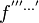$f^{'''\dots'}$ where${}^'$ occurs a total of$k$ times, is defined as the function obtained by differentiating$f$ a total of$k$ times (i.e., taking the derivative, then taking the derivative of that, and so on, a total of$k$ times). The first few cases are shown explicitly:

Value of$k$ Notation with repeated primes for$f^{(k)}$$f^{(k)}$ notation Definition In words
0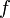$\! f$$\! f^{(0)}$$\! f$ the original function
1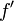$\! f'$$\! f^{(1)}$$\! f'$ the derivative, also called the first derivative
2$\! f''$$\! f^{(2)}$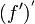$\! (f')^'$ the second derivative
3$\! f'''$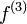$\! f^{(3)}$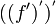$\! ((f')^')^'$ the third derivative

We could also define the$k^{th}$ derivative inductively as: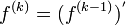$\! f^{(k)} = (f^{(k-1)})^'$

or as: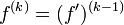$\! f^{(k)} = (f')^{(k-1)}$

with the base case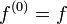$f^{(0)} = f$.

### Leibniz notation

Suppose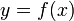$y = f(x)$, so$y$ is a dependent variable depending on$x$, the independent variable. The$k^{th}$ derivative of$y$ with respect to$x$ is denoted: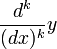$\! \frac{d^k}{(dx)^k}y$

or as:$\! \frac{d^ky}{dx^k}$

and is defined as: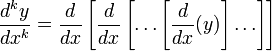$\! \frac{d^ky}{dx^k} = \frac{d}{dx}\left[\frac{d}{dx} \left[ \dots \left[\frac{d}{dx}(y)\right] \dots \right] \right]$

where the$d/dx$ occurs$k$ times. Alternatively we can define it inductively as: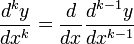$\! \frac{d^ky}{dx^k} = \frac{d}{dx} \frac{d^{k-1}y}{dx^{k-1}}$

with the base case$k = 0$ being defined as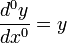$\frac{d^0y}{dx^0} = y$.